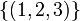# Pronormal not implies join with any distinct conjugate is the whole group

This article gives the statement and possibly, proof, of a non-implication relation between two subgroup properties. That is, it states that every subgroup satisfying the first subgroup property (i.e., pronormal subgroup) need not satisfy the second subgroup property (i.e., subgroup whose join with any distinct conjugate is the whole group)
View a complete list of subgroup property non-implications | View a complete list of subgroup property implications
Get more facts about pronormal subgroup|Get more facts about subgroup whose join with any distinct conjugate is the whole group
EXPLORE EXAMPLES YOURSELF: View examples of subgroups satisfying property pronormal subgroup but not subgroup whose join with any distinct conjugate is the whole group|View examples of subgroups satisfying property pronormal subgroup and subgroup whose join with any distinct conjugate is the whole group

## Statement

We can have a pronormal subgroup$H$ of a group$G$, and a conjugate subgroup$K \ne H$ of$H$ in$G$ such that$\langle H, K \rangle$ is not the whole group$G$.

## Facts used

1. Sylow implies pronormal

## Proof

### Example of the symmetric group of degree four

Further information: symmetric group:S4

Suppose$G$ is the symmetric group on$\{ 1,2,3,4 \}$. Let$H$ be the$3$-Sylow subgroup generated by$\{ (1,2,3) \}$. Then, by fact (1), we get that$H$ is pronormal in$G$. On the other hand, the subgroup generated by$H$ and its conjugate subgroup$\langle (1,3,4) \rangle$ is the alternating group on$\{ 1,2,3,4 \}$, which is a proper subgroup of$G$.